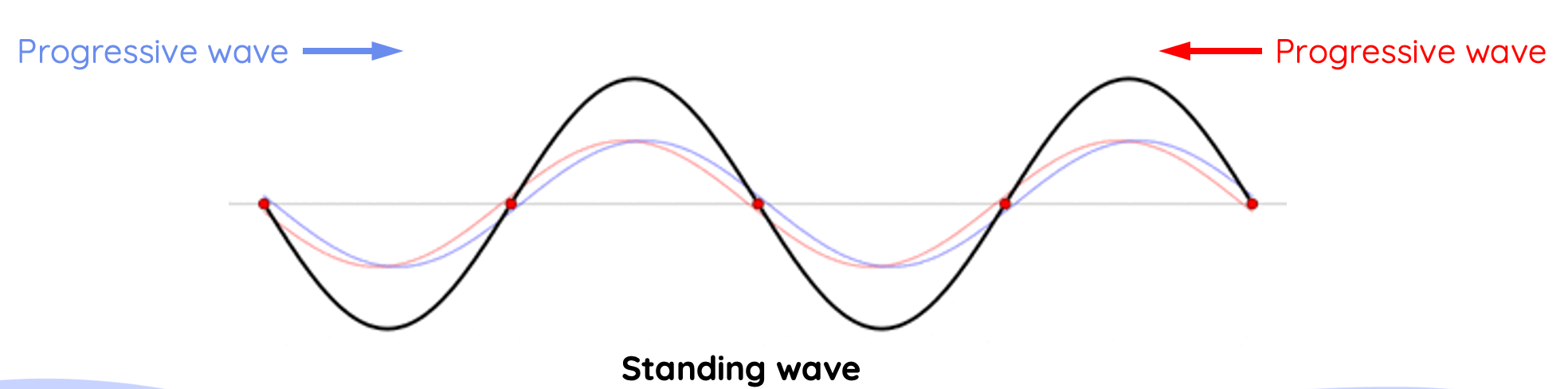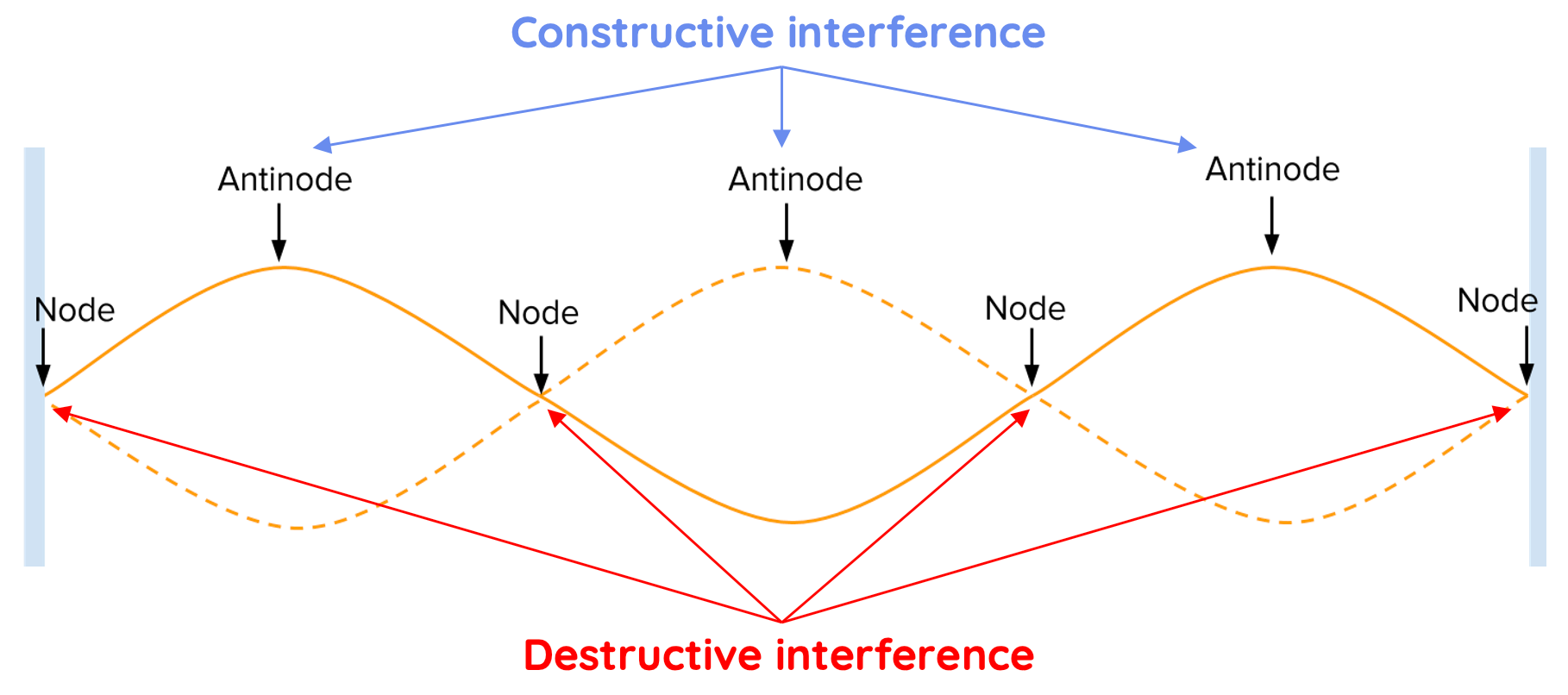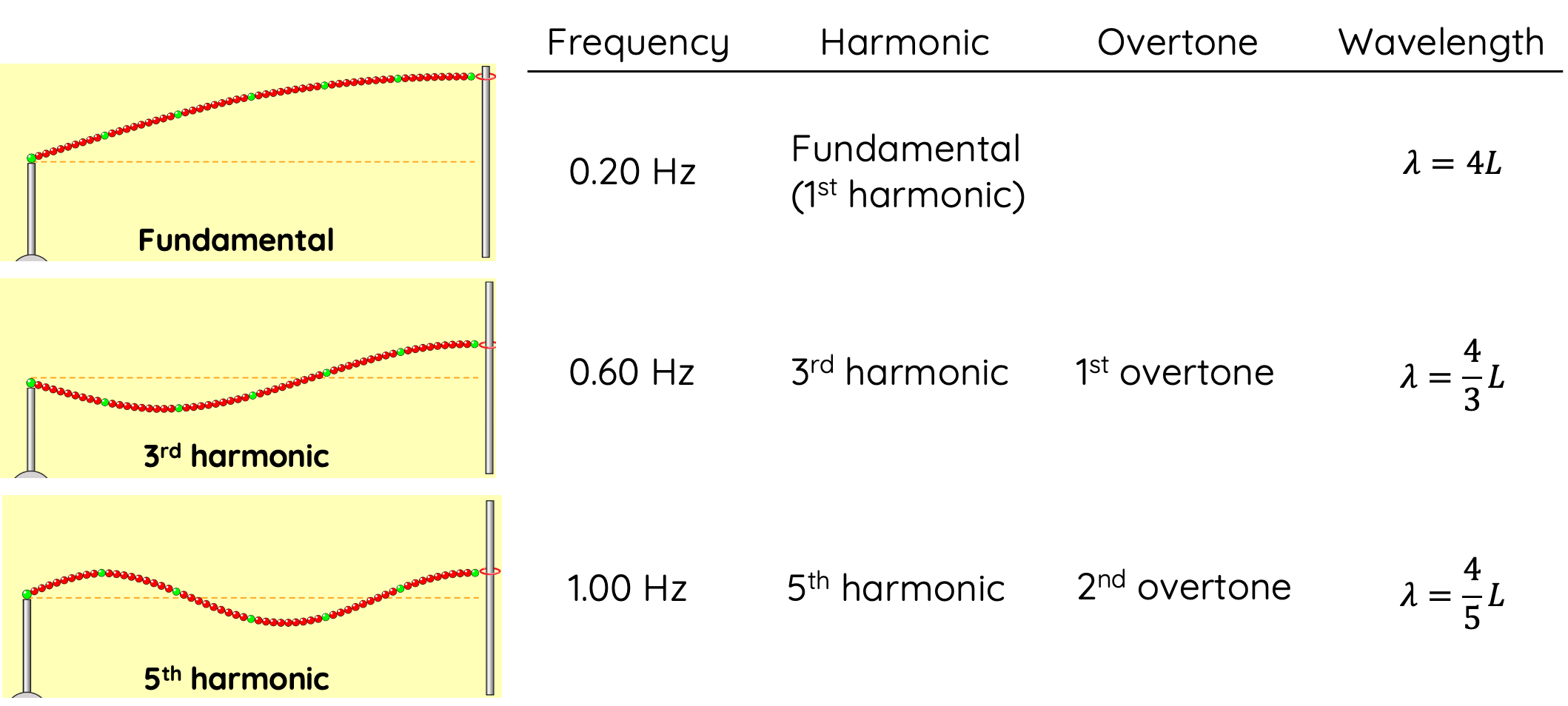# Progressive and Standing Waves

This topic is part of the HSC Physics course under the section Wave Behaviour.

### HSC Physics Syllabus

• conduct an investigation to distinguish between progressive and standing waves (ACSPH072)
• investigate and model the behaviour of standing waves on strings and/or in pipes to relate quantitatively the fundamental and harmonic frequencies of the waves that are produced to the physical characteristics (eg length, mass, tension, wave velocity) of the medium (ACSPH072)

### Progressive and Standing Waves: Delving into Wave Physics

Waves play an integral role in our everyday life, from the sounds we hear to the light we see. To develop a comprehensive understanding of wave behaviour, it's essential to distinguish between progressive and standing waves. Let's embark on a journey to explore these fascinating wave phenomena.

### What are Progressive Waves?

Also known as travelling waves, progressive waves carry energy from one point to another without the medium itself moving. Picture the ripples created when a stone is thrown into a pond - they move outward, transferring energy as they go.

#### Characteristics of progressive waves:

• Transmission of Energy: These waves transfer energy and momentum through the medium.

• Directional Movement: They have a clear direction of propagation, moving from the source to other parts of the medium.

• Examples: Sound waves travelling through air or ripples moving across the water surface.

### What are Standing Waves?

Contrary to progressive waves, standing waves, or stationary waves, don't travel or propagate. They form when two waves of the same frequency interfere while moving in opposite directions. The resultant wave appears to "stand still", that is, it continues to oscillate but does not propagate from one place to another.

#### Characteristics of standing waves:

• No Net Energy Transmission: While energy oscillates between the wave's potential and kinetic forms, it doesn't move forward with the wave.

• Nodes and Antinodes: Key features of standing waves. Nodes are points of no displacement, while antinodes are points of maximum displacement.

• Formation: Typically formed due to the reflection of a wave in a confined space, such as a guitar string fixed at both ends.

### How are Standing Waves Formed?

Standing waves are typically formed due to the interference of two waves with the same frequency, travelling in opposite directions within a bounded or confined medium.This interference can be a result of:

• Reflection: When a wave reflects off a boundary or barrier and interferes with incoming waves of the same kind. For example, a wave moving down a string reflects back upon reaching a fixed end, creating a standing wave.

• Opposing Wave Sources: Two coherent wave sources (with the same frequency and phase) placed such that they send waves towards each other can produce standing waves as their waves interfere.

For standing waves to form, certain conditions need to be met:

• Boundary Conditions: The medium must be bounded or restricted in some manner, either being confined between two points (like a string fixed at both ends) or reflecting waves back (like an echo in a closed room).

• Matching Frequencies: The interfering waves should have the same frequency. This ensures that their interference results in a stable, stationary pattern of nodes and antinodes.

• Stable Phase Relationship: The waves should maintain a stable phase relationship, meaning they should remain in-phase or out-of-phase consistently.

The following video shows the formation of a standing wave (black) from two progressive waves of the same frequency travelling in the opposite direction. Note that the standing wave oscillates, but does not seem to move to the left nor the right.

Waves do not need to have the same amplitude to produce a standing wave. However, when their amplitudes are equal, the resultant standing wave is the most pronounced, and this is more commonly seen.

### What are Antinodes and Nodes?

Antinodes and nodes of a standing waves are the result of superposition of progressive waves.

• Constructive Interference: At some points, the two waves add up to produce areas of maximum displacement, known as antinodes.

• Destructive Interference: At other points, they cancel each other out, resulting in areas of no displacement, called nodes.

This alternating pattern of nodes and antinodes is a hallmark of standing waves.### Comparing Progressive and Standing Waves

Criteria Progressive Wave Standing Wave
Energy Movement Transfers energy No net transfer of energy
Direction Travels in one direction Doesn't travel
Examples Sound waves, light waves Guitar strings, drumheads

### Standing Waves on Strings

Standing waves can be produced by the reflection of a progressive wave in a string system that is either fixed or free to move at one end. The frequency of such a progressive wave must be specific to produce the standing wave for reasons we discussed above.

Access the standing wave on string simulation here

### Standing Wave On A String With A Fixed End

When a progressive wave in a string is reflected off a fixed end, it experiences a 180º phase change. If the original progressive wave has a positive amplitude, then the reflected wave has a negative amplitude.

The lowest frequency required to produce a standing wave is the fundamental frequency, f_0, also called the first harmonic.

Frequencies required to form the standing wave are integral multiples of the fundamental frequency:

$$f = nf_0 \, \, \text{where n = 1, 2, 3...}$$

The frequency twice the fundamental frequency is called the second harmonic. This is also the first frequency after the fundamental frequency that can produce a standing wave, hence its alternative name, first overtone.

The next frequency (three times the fundamental) is called the third harmonic and second overtone.The wavelength of the standing wave decreases as its frequency increases such that:

$$\lambda = \frac{2}{n}L \, \, \text{where n = 1, 2, 3...}$$

where L is the length between two ends of the string.

Standing waves form in a pipe with both ends open such that an antinode (point at which maximum displacement occurs) if present at each open end. The number of nodes (points at which there are no oscillation) also corresponds to the harmonic number (n). For example, a second harmonic standing wave has two nodes.

### Standing Wave On A String With Free To Move End

When a progressive wave is reflected off an end that is not fixed but free to move, it does not experience a phase change. This means if the original progressive wave has a positive amplitude, the reflected wave will also have a positive amplitude. This difference changes the frequency required to form a standing wave in a string which as a free to move end.

Frequencies required to form a standing wave in this system are given by

$$f = nf_0 \, \, \text{where n = 1, 3, 5...}$$

Note that must be an odd integer when one end of the string is free to move.

The first frequency after the fundamental frequency is the third harmonic, which is three times the fundamental frequency.

This also means there are no second (n = 2), fourth (n = 4) harmonics for standing waves in this system.The wavelength of standing waves is given by

$$\lambda = \frac{4}{n}L \, \, \text{where n = 1, 3, 5...}$$

where L is the length between two ends of the string.

### Standing Waves in Pipes

The production of standing waves using sound in pipes and tubes is discussed separately here